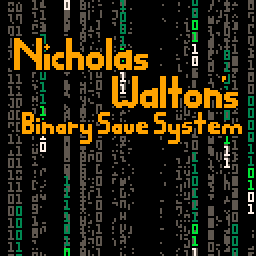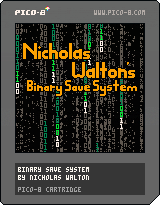##### Subscribe to the Croaking Kero NewsletterPico-8 has a simple save system which allows you to set a cartridge ID: `cartdata("mycoolgame")` Then store/read 64 number values, each 32 bits: ```dset(index, value) var = dget(index)``` This works well enough if all you want to store is the level a player has reached and a high score. But what if you want to store more? The simplest way to expand this storage is by peeking and poking the memory directly. After running cartdata as above you can access your saved data in memory from 0x5e00 to 0x5eff ```value = peek(0x5e00) poke(0x5e00, value)``` Peek and poke read and write a single byte (8 bits), so by using these you can quadruple the number of values you can store! However these values are limited to 8 bit integers. You can mix and match 32-bit and 8-bit numbers by also utilizing peek4 and poke4 which are the same as peek and poke but use 4 bytes each instead of 1. However, what if 256 8-bit numbers aren’t enough for you? What if, hypothetically, you were working on a Pokemon-inspired monster-catching RPG, having to store the level, selected moves and experience for each mon in your party, and keeping track of the players items, having a customizable character and multiple save slots? Well then you’d probably need a binary save system. The binary save system I’ve come up with works like this: - Start with a binary table of 2048 boolean (true/false) values. - Convert various kinds of data to binary and store it in the table. - Convert the table to 8-bit chunks. - Save/load these 8-bit chunks with peek/poke. With the above system you can store any kind of data you want, as long as you can convert it to and from binary. Booleans are simple; you set a value in the binary table: `bintable[n] = true` Now the first thing to do is to figure out how we can take our binary table and save it into the cartdata, and load that cartdata back into the binary table. Let’s start with saving. ```function commit_bintable() for i=0,127 do poke(0x5e00+i, get_poker(i)) end end``` This function is pretty simple. It iterates 128 times and pokes 128 “pokers” into the cartdata. The tougher part is creating those pokers. Here’s the code: ```function get_poker(_i) _i=_i*8+1 local _poker=0 for n=0,7 do if(bintable[_i+n]) _poker += 2^n end return _poker end``` First we take our _i value (0-127) which is the poker index and convert it to a starting position in the binary table. Then we loop over 8 bits in the table to calculate _poker. Starting from bit 1 and going to bit 8 in the table, each bit represents 2^n. So to switch the nth bit from 0 to 1 in our integer poker we add 2^n to it. This results in some meaningless number whose bits each correspond to the 8 bits in this section of the binary table. ```Binary: 0 0 0 0 0 0 0 0 Represent: 1 2 4 8 16 32 64 128``` By adding any of those 2^n numbers we can switch a 0 bit to a 1. And that’s saving the binary table done! On to loading it: ```function load_bintable() for i=0,127 do local _poker=peek(0x5e00+i) for j=0,7 do bintable[i*8+1+j] = get_bit(_poker,j) end end end``` We iterate over our 128 “pokers” – though we’re peeking them this time – and extract each of their 8 bits into the binary table. The key to all this is the get_bit function. So here it is: ```function get_bit(_value,_n) return flr(shr(_value,_n))%2 == 1 end``` Let’s break that down. We take our _value which in this case will be an 8-bit integer. We shift its bits _n spaces to the right, remove everything after the decimal point and check whether it’s even or odd. Sound a bit complicated? Let’s take a look at what’s happening in binary. Say we’ve retrieved a poker and it’s 203. In binary: `0b11001011` Then we shift the bits to the right 1 space: `0b1100101.1` We no longer have an integer so we floor it: `0b1100101` Then we use the % (modulus) operator to check if 2 divides into it evenly or if there is a remainder. If the rightmost bit is 1 then the number is odd and our value%2 will return 1. If the rightmost bit is 0 then the number is even and value%2 will return 0. To convert this to a boolean value (true/false) we compare it with ==1 and return the result. Just like that we can take our 8-bit pokers and extract each bit into our binary table! Now that we’ve got a binary table being saved and loaded let’s fill it with useful information! The most important thing to start with is integers. I want each integer to take up the minimum number of bits necessary for that particular piece of data so I’ve created these functions: ```function numtobintable(_value,_dest,_nbits) for i=0,_nbits-1 do bintable[_dest+i]=get_bit(_value,i) end end function bitstonum(_addr,_nbits) local _p=0 for i=0,_nbits-1 do if(bintable[_addr+i])_p+=2^i end return _p end``` In numtobintable we take an integer value, destination in the binary table and a number of bits to take up. All we have to do is iterate over each bit in the number with get_bit and put those bits into the binary table. Easy! Loading the integers is a little more difficult but it should be familiar. We start with the number 0 then iterate over each bit, adding 2^i whenever the bit is true and not adding anything for false. Pretty simple really, aye? But hold on, how do you know how many bits to use for each integer? You’ll have to figure out what the maximum value is you might be saving for each variable, then find the lowest power of 2 number it fits into, minus 1. With a single bit you can store 2 values, with 2 bits you can store 4, then 8, 16, etc. Say you want to save a Notemon’s level for example. Notemon can be any level from 1 to 50. That’s 50 values, so the lowest power of 2 greater than or equal to that is 64. 64 is 2^7. Minus 1 equals 6, so we need 6 bits to store values from 1-50. To verify, our 6 bits will represent multiples of 1, 2, 4, 8, 16, 32. If all of those bits were true they’d add up to 63. Including 0 as a possible value that means 64 total values so we’ve found the minimum number of bits necessary to save this piece of information! So with that we can store useful information in our binary table. That really is all you need to make a pretty comprehensive save/load system! One last thing before I sign off from my first article though: strings! I’m not currently using this feature in Notemon, but at one point I wanted players to be able to name their mon so I made the following code: ```chartoint={a=1,b=2,c=3,d=4,e=5,f=6,g=7,h=8,i=9,j=10, k=11,l=12,m=13,n=14,o=15,p=16,q=17,r=18,s=19,t=20, u=21,v=22,w=23,x=24,y=25,z=26,_=27} inttochar={"a","b","c","d","e","f","g","h","i","j", "k","l","m","n","o","p","q","r","s","t", "u","v","w","x","y","z","_"} function save_string(_str,_addr,_len) for i=0,_len-1 do numtobintable(27,_addr+i*5,5) end for i=1,#_str do numtobintable(chartoint[sub(_str,i,i)],_addr+(i-1)*5,5) end end function load_string(_addr,_l) local _s="" for i=0,_l-1 do local _c=inttochar[bitstonum(_addr+i*5,5)] if(_c=="_"or _c==nil)break _s=_s.._c end return _s end``` chartoint and inttochar aren’t even functions – they’re tables! Since Pico-8 doesn’t have any ord() function to convert characters to and from integers I just made a couple of tables to go back and forth. Simple stuff. save_string has two for loops which might seem odd at first. The first loop starts at the address and basically clears all the characters to the “_” character which I chose as a code meaning “end string here”. It could be anything, or you could have fixed length strings and not need this first loop but Notemon names needed to be variable in length. Next we loop over each character in the string and add it to the binary table. See all that *5 stuff going on? That’s because I allowed for 27 characters. As above I calculated that would require 5 bits to store. In load_string we start with an empty string then copy each character from the binary table into the string until we hit the “_” character. That’s all, folks! I really hope this is helpful to the Pico-8 community. I have previously searched for a Pico-8 binary save system and found something on the BBS but it was esoteric. Instead I muddled my way until I finally understood binary and how to use it in Pico-8. Here’s a cart with all the code, along with some bonus code for the naming screen I made for Notemon and the code to generate that matrix background you see in the cart image 🙂Thanks for reading my post. Expect more Pico-8 tech posts as I continue creating Notemon! Also available on the Pico-8 BBS: https://www.lexaloffle.com/bbs/?tid=30711